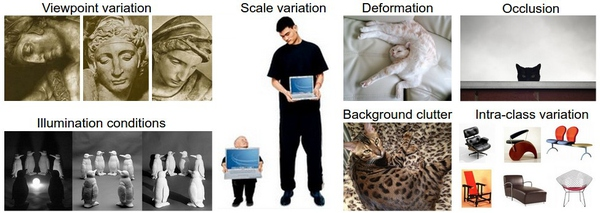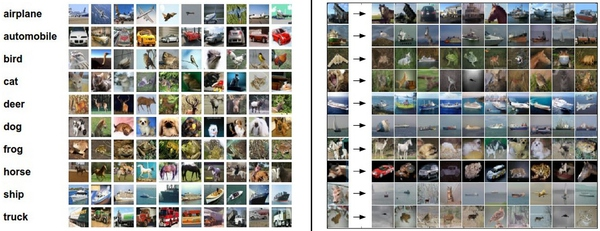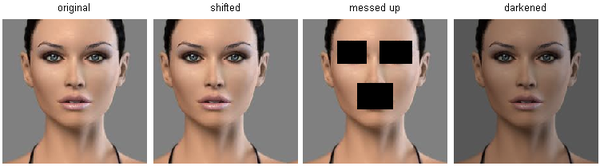# 计算机视觉之图像分类基础

Posted by jjx on February 23, 2017

• 视角变化（Viewpoint variation）：同一个物体，摄像机可以从多个角度来展现。
• 大小变化（Scale variation）：物体可视的大小通常是会变化的（不仅是在图片中，在真实世界中大小也是变化的）。
• 形变（Deformation）：很多东西的形状并非一成不变，会有很大变化。
• 遮挡（Occlusion）：目标物体可能被挡住。有时候只有物体的一小部分（可以小到几个像素）是可见的。
• 光照条件（Illumination conditions）：在像素层面上，光照的影响非常大。
• 背景干扰（Background clutter）：物体可能混入背景之中，使之难以被辨认。
• 类内差异（Intra-class variation）：一类物体的个体之间的外形差异很大，比如椅子。这一类物体有许多不同的对象，每个都有自己的外形。
• 面对以上所有变化及其组合，好的图像分类模型能够在维持分类结论稳定的同时，保持对类间差异足够敏感。• 输入：输入是包含N个图像的集合，每个图像的标签是K种分类标签中的一种。这个集合称为训练集。
• 学习：这一步的任务是使用训练集来学习每个类到底长什么样。一般该步骤叫做训练分类器或者学习一个模型。
• 评价：让分类器来预测它未曾见过的图像的分类标签，并以此来评价分类器的质量。我们会把分类器预测的标签和图像真正的分类标签对比。毫无疑问，分类器预测的分类标签和图像真正的分类标签如果一致，那就是好事，这样的情况越多越好。

#### Nearest Neighbor分类器$d_1(I_1,I_2)=\sum(I_1^p-I_2^p)$

Xtr, Ytr, Xte, Yte = load_CIFAR10('data/cifar10/') # a magic function we provide
# flatten out all images to be one-dimensional
Xtr_rows = Xtr.reshape(Xtr.shape, 32 * 32 * 3) # Xtr_rows becomes 50000 x 3072
Xte_rows = Xte.reshape(Xte.shape, 32 * 32 * 3) # Xte_rows becomes 10000


nn = NearestNeighbor() # create a Nearest Neighbor classifier class
nn.train(Xtr_rows, Ytr) # train the classifier on the training images and labels
Yte_predict = nn.predict(Xte_rows) # predict labels on the test images
# and now print the classification accuracy, which is the average number
# of examples that are correctly predicted (i.e. label matches)
print 'accuracy: %f' % ( np.mean(Yte_predict == Yte) )


import numpy as np

class NearestNeighbor(object):
def __init__(self):
pass

def train(self, X, y):
""" X is N x D where each row is an example. Y is 1-dimension of size N """
# the nearest neighbor classifier simply remembers all the training data
self.Xtr = X
self.ytr = y

def predict(self, X):
""" X is N x D where each row is an example we wish to predict label for """
num_test = X.shape
# lets make sure that the output type matches the input type
Ypred = np.zeros(num_test, dtype = self.ytr.dtype)

# loop over all test rows
for i in xrange(num_test):
# find the nearest training image to the i'th test image
# using the L1 distance (sum of absolute value differences)
distances = np.sum(np.abs(self.Xtr - X[i,:]), axis = 1)
min_index = np.argmin(distances) # get the index with smallest distance
Ypred[i] = self.ytr[min_index] # predict the label of the nearest example

return Ypred


distances = np.sqrt(np.sum(np.square(self.Xtr - X[i,:]), axis = 1))


L1和L2比较。比较这两个度量方式是挺有意思的。在面对两个向量之间的差异时，L2比L1更加不能容忍这些差异。也就是说，相对于1个巨大的差异，L2距离更倾向于接受多个中等程度的差异。L1和L2都是在p-norm常用的特殊形式。

k-Nearest Neighbor分类器

k-NN分类器需要设定k值，那么选择哪个k值最合适的呢？我们可以选择不同的距离函数，比如L1范数和L2范数等，那么选哪个好？还有不少选择我们甚至连考虑都没有考虑到（比如：点积）。所有这些选择，被称为超参数（hyperparameter）。在基于数据进行学习的机器学习算法设计中，超参数是很常见的。一般说来，这些超参数具体怎么设置或取值并不是显而易见的。

# assume we have Xtr_rows, Ytr, Xte_rows, Yte as before
# recall Xtr_rows is 50,000 x 3072 matrix
Xval_rows = Xtr_rows[:1000, :] # take first 1000 for validation
Yval = Ytr[:1000]
Xtr_rows = Xtr_rows[1000:, :] # keep last 49,000 for train
Ytr = Ytr[1000:]

# find hyperparameters that work best on the validation set
validation_accuracies = []
for k in [1, 3, 5, 10, 20, 50, 100]:

# use a particular value of k and evaluation on validation data
nn = NearestNeighbor()
nn.train(Xtr_rows, Ytr)
# here we assume a modified NearestNeighbor class that can take a k as input
Yval_predict = nn.predict(Xval_rows, k = k)
acc = np.mean(Yval_predict == Yval)
print 'accuracy: %f' % (acc,)

# keep track of what works on the validation set
validation_accuracies.append((k, acc))


#### Nearest Neighbor分类器的优劣

Nearest Neighbor分类器在某些特定情况（比如数据维度较低）下，可能是不错的选择。但是在实际的图像分类工作中，很少使用。因为图像都是高维度数据（他们通常包含很多像素），而高维度向量之间的距离通常是反直觉的。下面的图片展示了基于像素的相似和基于感官的相似是有很大不同的：